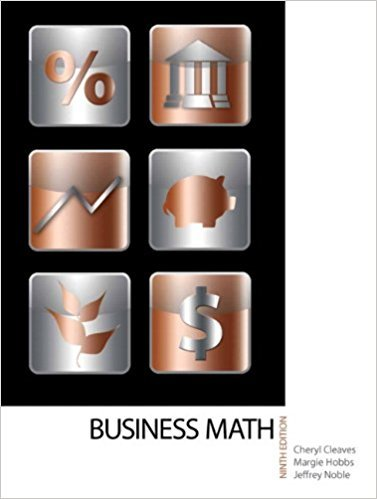×
×

# Find the cost of ending inventory and the cost of goods sold using the tables inISBN: 9780135108178 355

## Solution for problem 5 Chapter 18

• Textbook Solutions
• 2901 Step-by-step solutions solved by professors and subject experts
• Get 24/7 help from StudySoup virtual teaching assistants4 5 1 326 Reviews
12
2
Problem 5

Find the cost of ending inventory and the cost of goods sold using the tables in Exercises 1 and 2.

Step-by-Step Solution:
Step 1 of 3

th Math 340 Lecture – Introduction to Ordinary Differential Equations – April 25 , 2016 What We Covered: 1. Prepare for Quiz (second to last one guys!) a. This will cover 4.5 and 5.1 2. Course Content – Chapter 5: The Laplace Transform (LT) a. Section...

Step 2 of 3

Step 3 of 3

##### ISBN: 9780135108178

Unlock Textbook Solution